6493

Physics Properties Of Matter Level: Misc Level

A large stone is resting on the bottom of the swimming pool. The normal force of the bottom of the pool on the stone

a. is equal to the weight of the stone

b. is equal to the weight of the water displaced

c. is equal to the sum of the weight of the stone and the weight of the displaced water

d. is equal to difference between the weight of the stone and the weight of the displaced water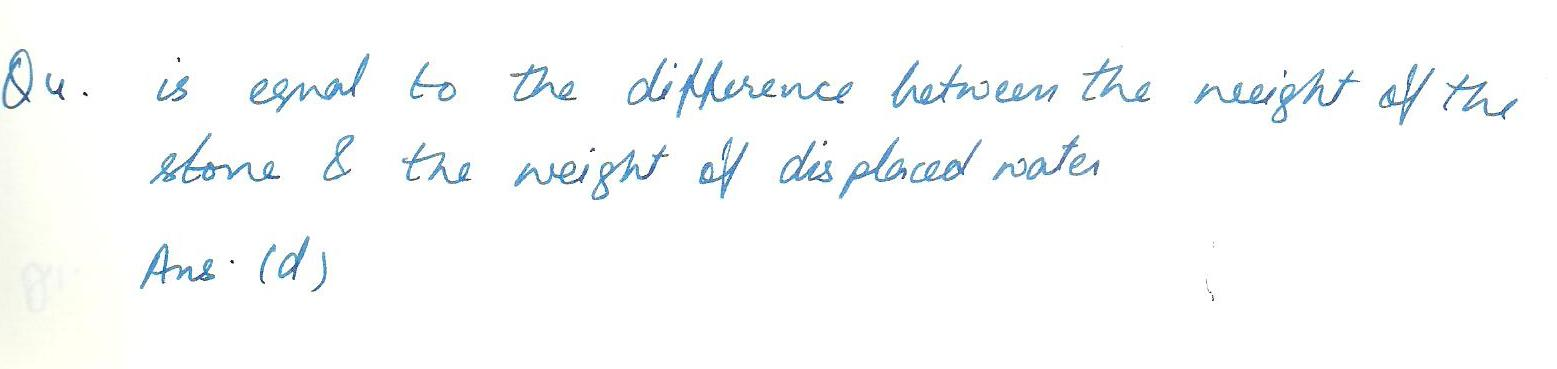6491

Physics Properties Of Matter Level: Misc Level

A glass container is half-filled with mercury, and a steel ball is floating on the mercury.If water is then poured on top of the steel ball and mercury, filling glass, what will happen to the steel ball?

a. If will float higher in the mercury

b. If will float at the same height.

c.It will float lower in the mercury.

d.It will sink to the bottom of the mercury.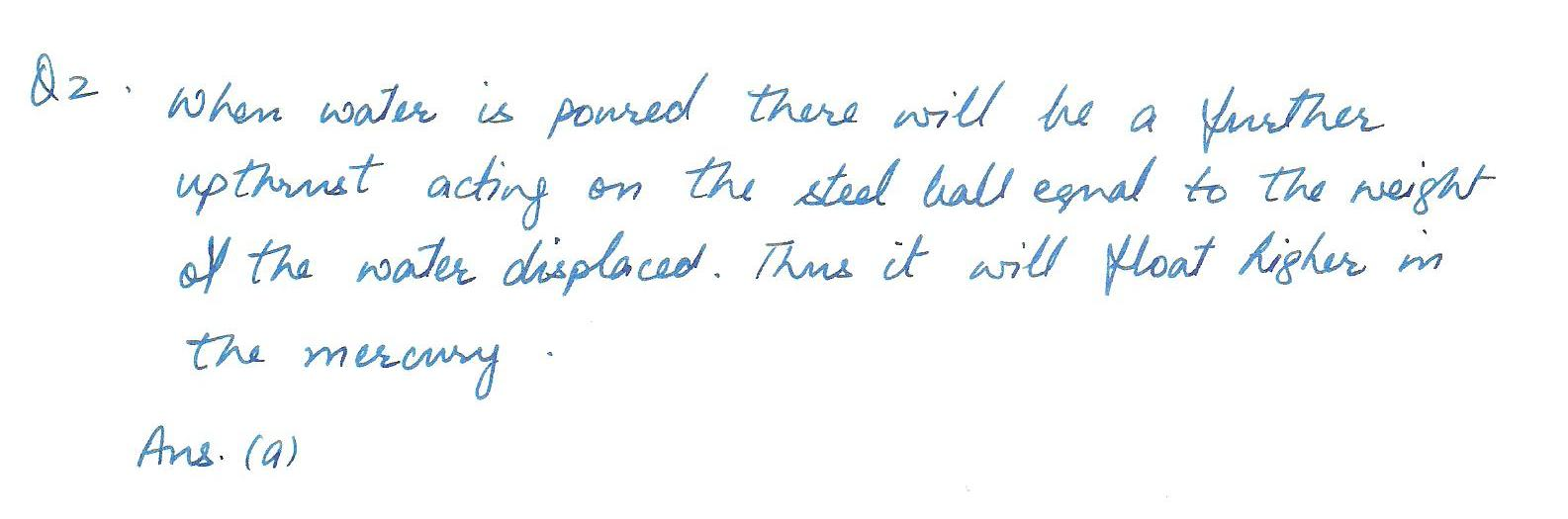6334

Physics Properties Of Matter Level: Misc Level

What mass of steam at a temperature of 100.% C, must be added to 2.9 kg of ice at a temperature of 0.0%C to yield liquid water having a temperature of 25.0%C?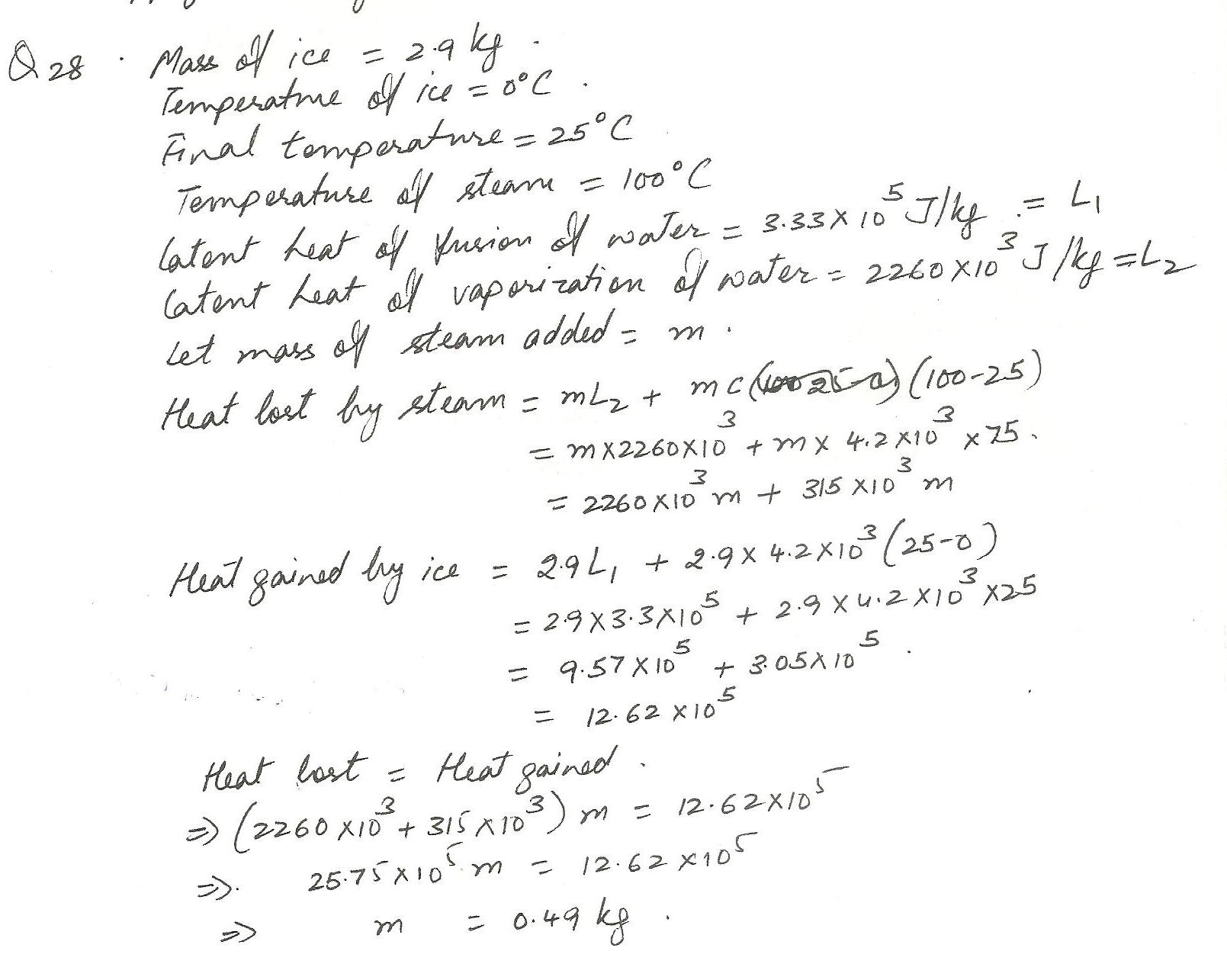6299

Physics Properties Of Matter Level: Misc Level

When the two arms of a U-tube manometer containing mercury are connected to two chambers, the difference in the heights of the mercury in the two arms is 4.5 cm. What is the difference in pressure between the chambers?(Density of mercury=13600 kg/m3 and g=9.8 m/s2)

a.6.0x105 Pa

b.1.0 x104 Pa

c.1.0 x105Pa

d. 6.0 x10 3 Pa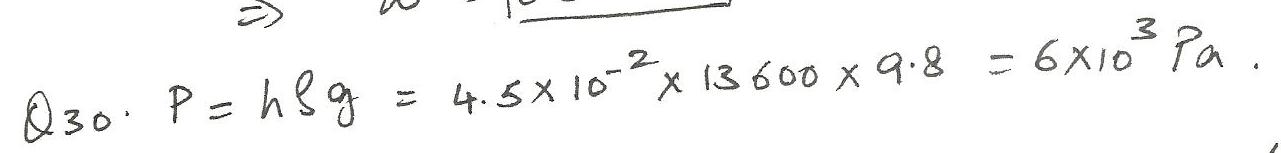6269

Physics Properties Of Matter Level: Misc Level

The specific heat of gas at constant pressure is 1.70 times the specific heat at constant volume. Find the specific heat at constant pressure. (Universal gas constant R=8.31 J/(mol K)

a. 20.2 J/ (mol K)

b.12.5 J/(mol K)

c.24.0 J/(mol K)

d. 18.0J(mol K)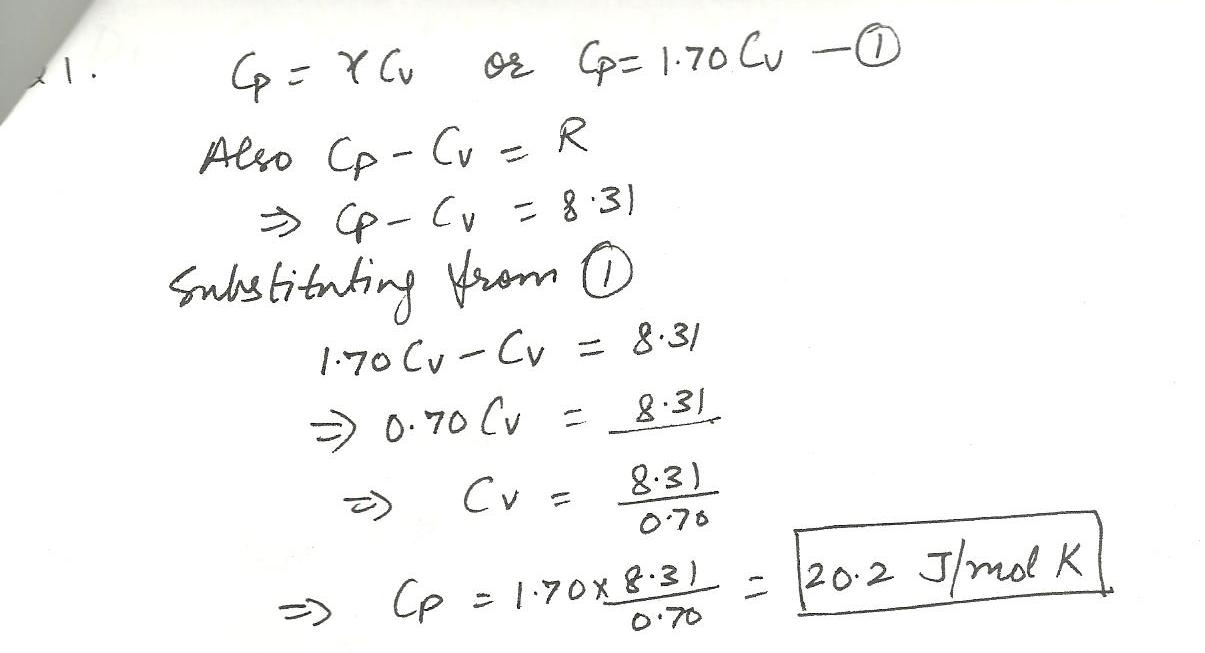6226

Physics Properties Of Matter Level: Misc Level

Water is pumped through a pipe to a tank at the top of a building. The pressure of the water at ground level is 2.2 x105 Pa and at the tank, the pressure is atmosopheric.If the diameter of the pipe is the same throughout, find the height at which the tank is located. Atmospheric pressure may be assumed as 1.0 x105 Pa and g as m/s2.

a. 24 m

b.16 m

c. 12 m

d. 20 m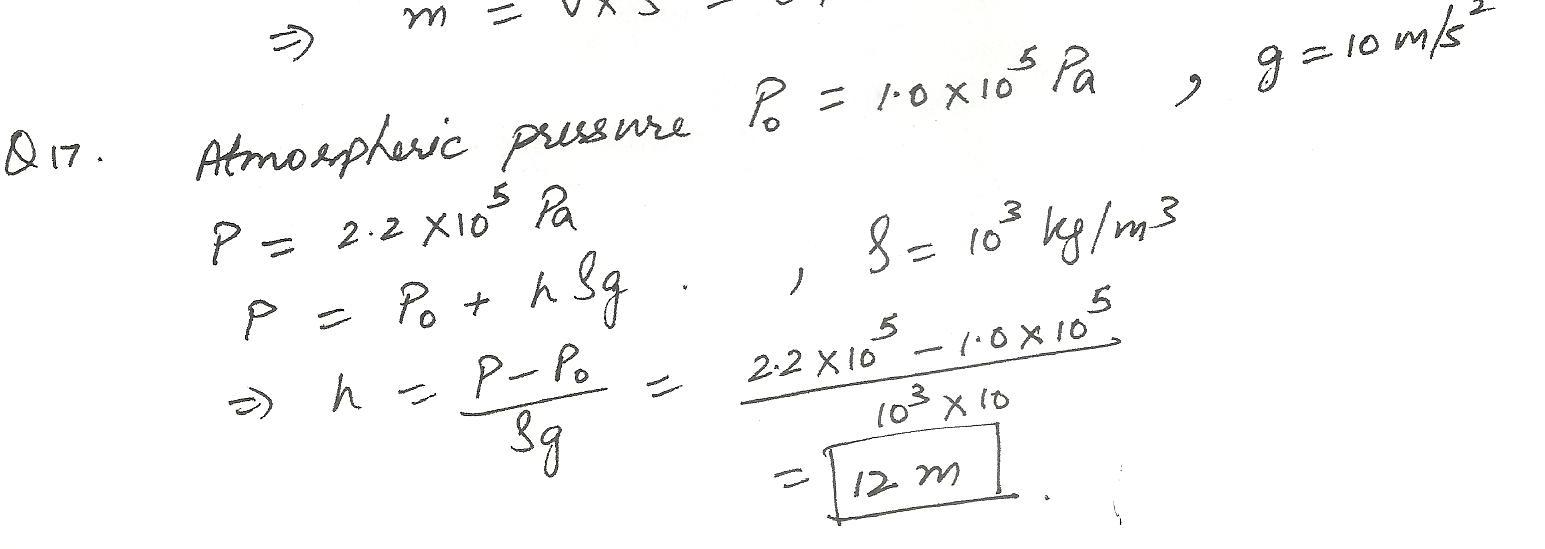6225

Physics Properties Of Matter Level: Misc Level

A log of wood is floating in water with 40% of its volume above the surface of the water.If the volume of the log is 0.4 m3, find the mass of the log.

a. 16 kg

b. 160 kg

c.240 kg

d. 200 kg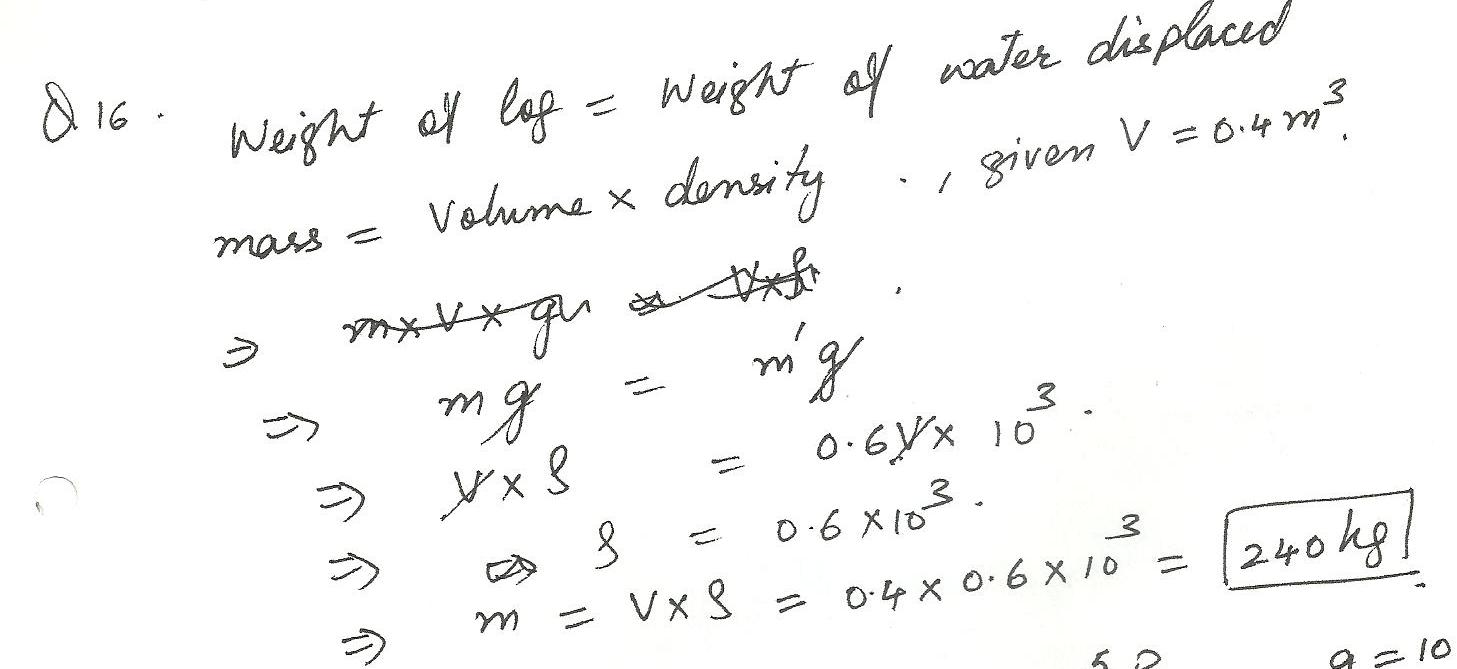6220

Physics Properties Of Matter Level: Misc Level

The gauge pressure at a depth of 17 m in equal to:

a. 1.7 x 103 Pa

b. 1.7 x105 Pa

c.1.7 x 104 Pa

d. 1.7 x 10 6 Pa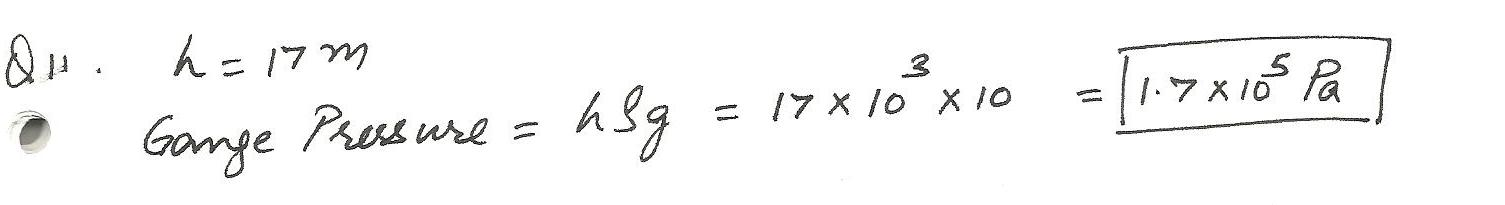6217

Physics Properties Of Matter Level: Misc Level

A force of 1.0x103 N is applied to a steel rod of length 1 m and a circular cross- section of area 10 cm2. Find the elongation of the rod. Young,s modulus for steel is 2.0 x 1011 Pa.

a. 2.0 x10-6 m

b. 3.0 x10-6m

c. 1.0 x10-6 m

d. 5.0 x 10-6m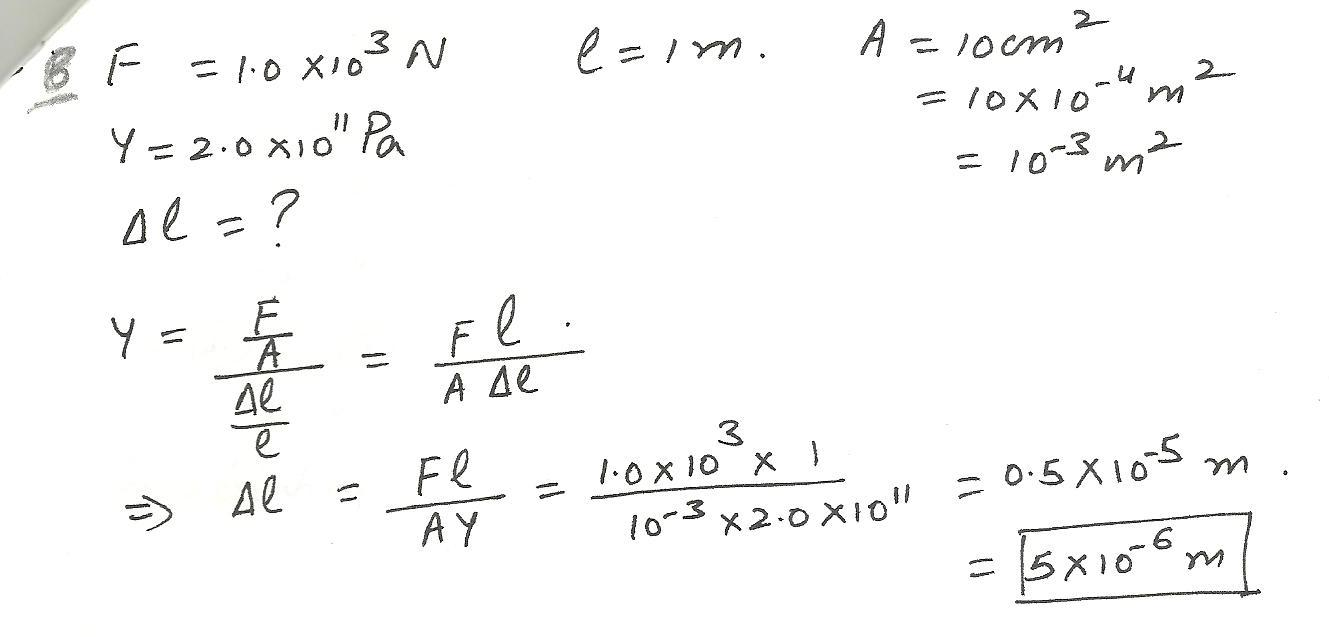6179

Physics Properties Of Matter Level: Misc Level

2 cubes of different mass and same density placed in water, what happens to displacement?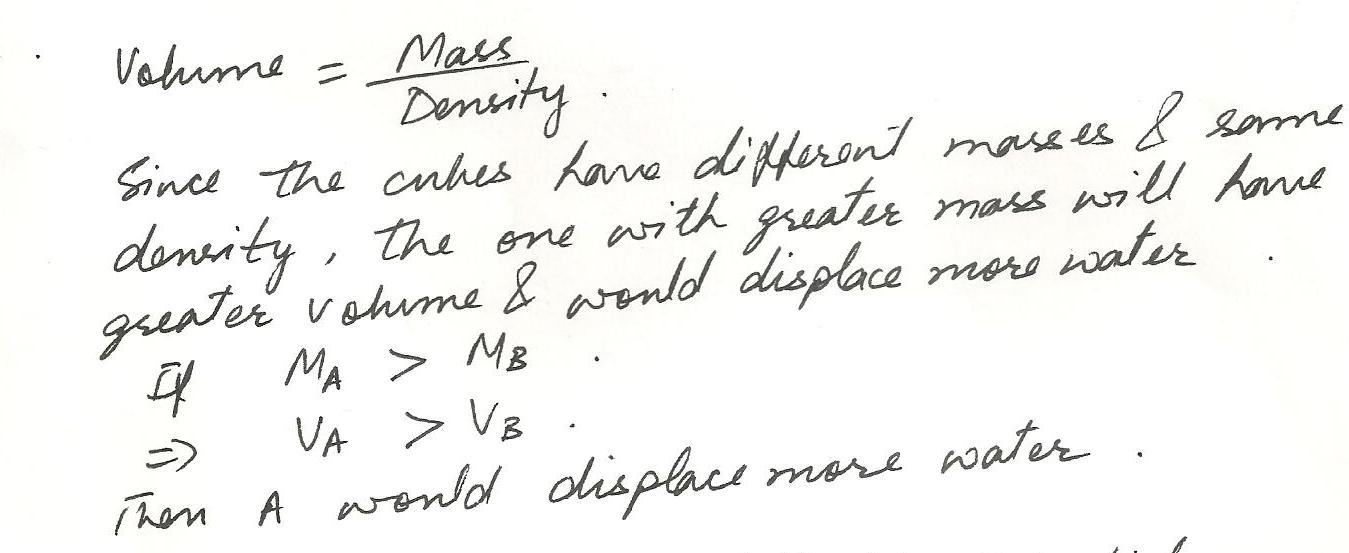6159

Physics Properties Of Matter Level: Misc Level

the pressure inside a gas bottle at 23.5 celcius is 3540 kPa. what will be pressure inside the bottle if 36% of the gas is released while the temperature drops to 8.8 celcius. (in kPa)

a)1.69 all answer power of 3 b) 1.91 c) 2.15 d) 2.43 e)2.75 f) 3.11 g) 3.51 h) 3.97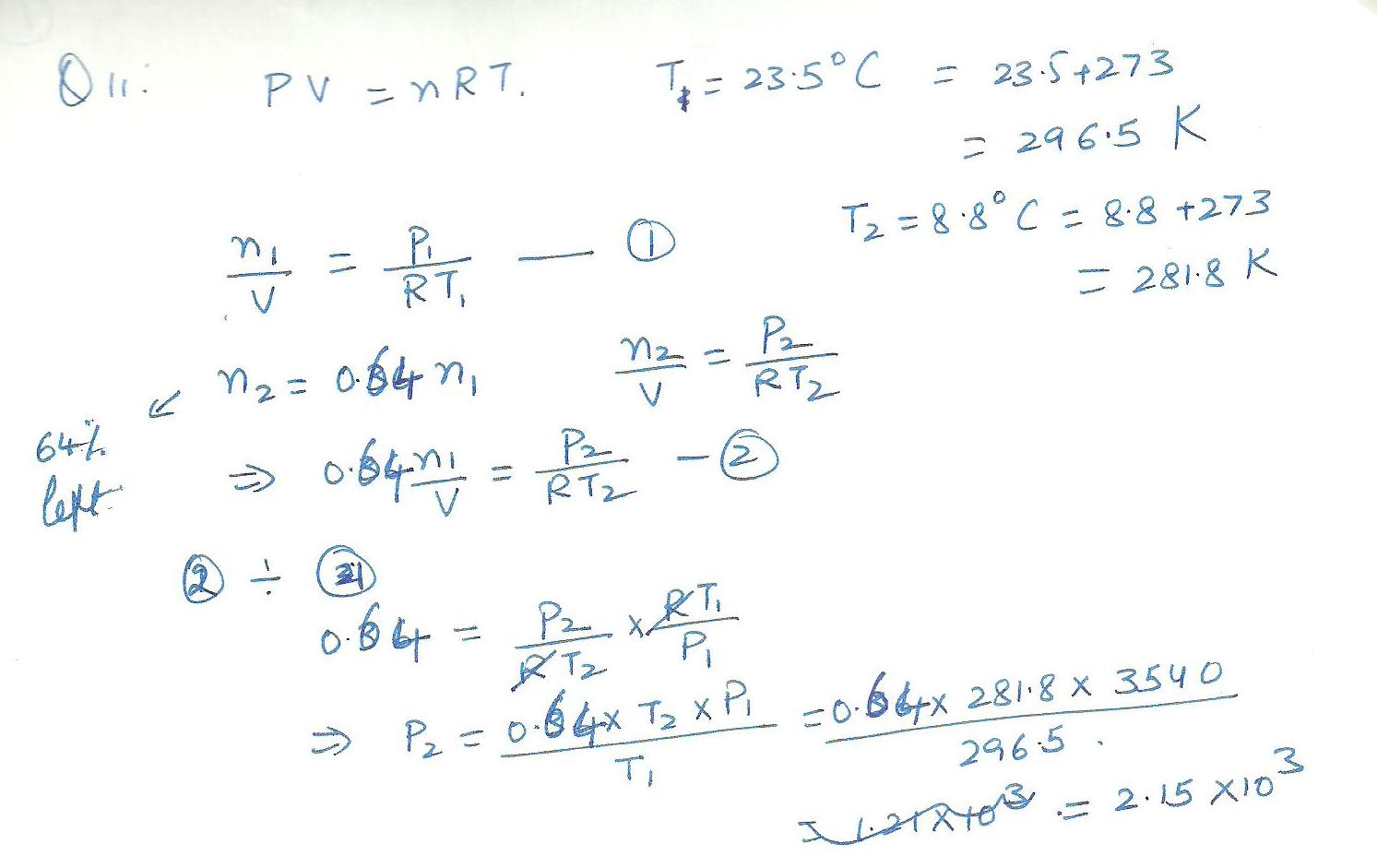6158

Physics Properties Of Matter Level: Misc Level

a piece of moon rock reads 43.6 grams on a scale when in air, but 20, 4 grams in a fluid having a specific gravity of 0.86. what is the density of the moon rock in kg/m^3?

a)4.24*10^2 b)5.30 power of 2 c)6.62 power of 2 d)8.27 power of 2 e)1.03 power of 3 f) 1.29 power of 3 g) 1.26 power of 3 h)2.02 power of 36157

Physics Properties Of Matter Level: Misc Level

the pressure at A is --the pressure at B

a) equal to b) greater than c) less than * the pictture is a bottle facing right with B being the skinny neck and A being the wide end end at the base*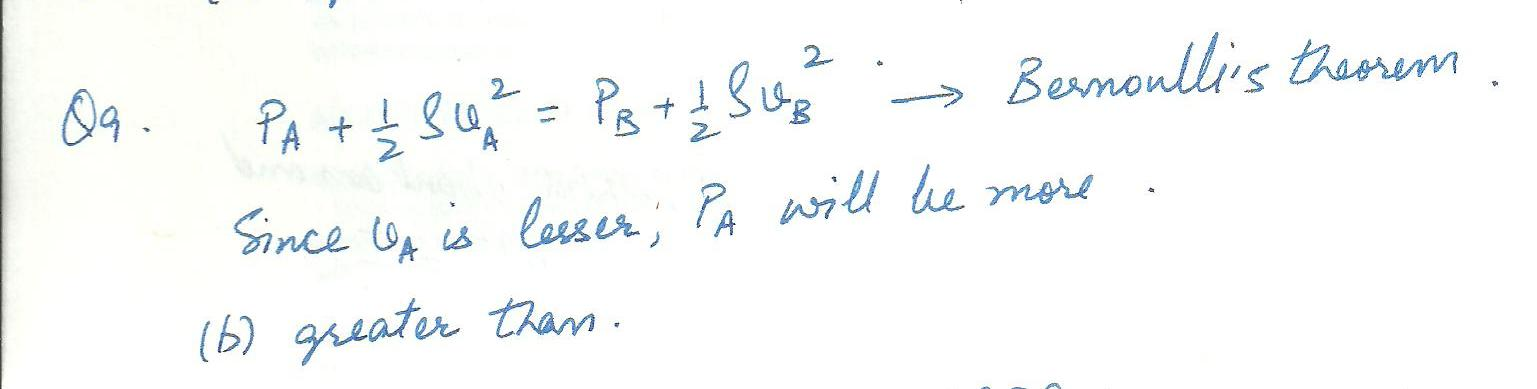6156

Physics Properties Of Matter Level: Misc Level

the amount of fluid that passes through A in one second is ---the amount of fluid that passes B an one second.

a) equal to b)greater than c)less than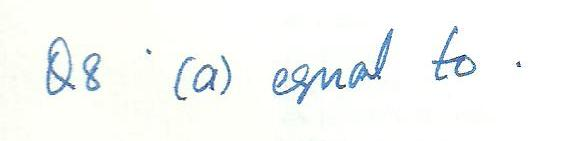6155

Physics Properties Of Matter Level: Misc Level

An incompressable fluid moves through the pipe shown above from right toleft. the pipde widens from the diameter of 3 cm at B to the diameter of 5 cm at A (Assume non viscous laminar flow.-the speed of the fluid at A is ---the speed of the fluid at B.

a) equal too b) greater than c) lessthan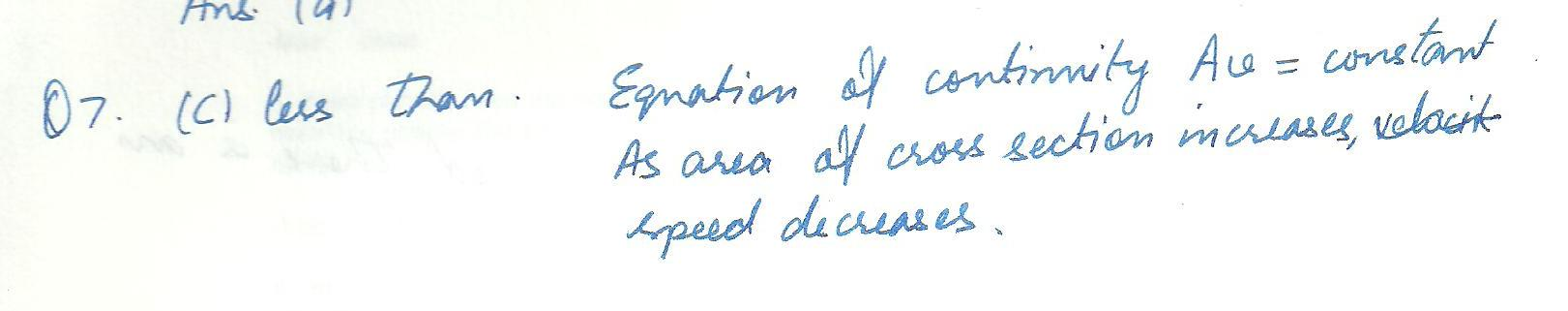Displaying 31-45 of 522 results.